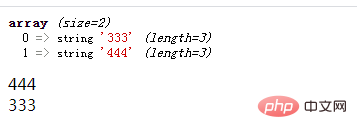# php怎么实现不使用第三个参数交换两个变量的值PHP中不用第三个变量交换两个变量的值

`<?php header("Content-type:text/html;charset=utf-8"); \$a=333; \$b=444; \$a=\$a+\$b; \$b=\$a-\$b; \$a=\$a-\$b; echo \$a."<br>"; echo \$b; ?>``<?php \$a=333; \$b=444; list(\$b,\$a)=array(\$a,\$b); echo \$a."<br>"; echo \$b; ?>``<?php \$a=333; \$b=444; \$b=explode("|", \$a."|".\$b); var_dump(\$b); \$a=\$b; \$b=\$b; echo \$a."<br>"; echo \$b; ?>`